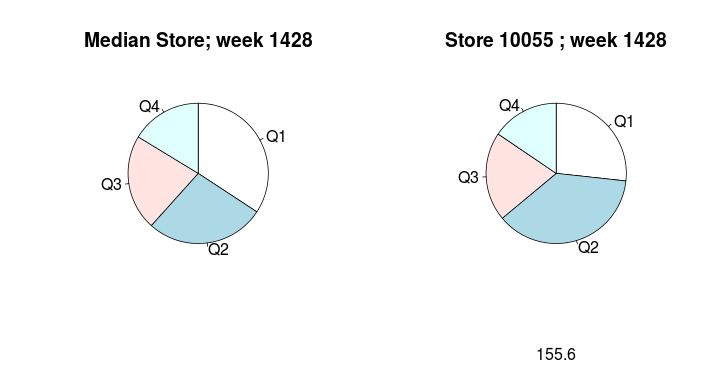Cours Stats

Abstract: The purpose of this study is to try to measure how store’s assortment differ from each other. Thus, one can explore where these differences come from, or how they relate to sales. This analysis uses retail panel data provided by IRI.

# Data and variables of interest

We first begin with sales data from the toothpaste category. It spans over nearly 150 stores and 12 weeks, and contains the weekly sales and prices of over 600 products (identified by their EAN).
The variables we keep to measure the differences between assortments are the price (actually the number of products per price quartiles), the proportion of private labels (numbre of PL products), and the type of products (e.g. toothpaste for children versus adults).

Price PL type
Q1 PL type1
Q2 NB type2
Q3 type3
Q4

For each value of these three variable, we calculate the market share in each assortment (store,week), e.g. :

$MS_{s,w,PQ1} = 100 \times \frac{Sales_{s,w,PQ1}}{\sum_{i}(Sales_{s,w,,PQi})}$

Then, we compare these Market Shares to the median of the corresponding Market Shares across all stores, e.g. :

$df_{s,w,PQ1} = \left( MS_{s,w,PQ1} - Med_{s} ( MS_{.,w,PQ1} ) \right)^{2}$

# Graphical result

Once these calculations are done, it is straightforward to select an assortment (e.g. s=100055, w=1428) and check the structure of its assortment again the median assortment (e.g. in terms of price quartiles):The graph also indicates the value of the df , the difference between the two assortments in terms of Market Shares.

Of course, this example is tipically a case where results would better fit into a shiny app. The one below let the user choose the category, the store, the week, and the variable to compare. Once these choices made, the app displays first the structure of the median store, the the structure of the chosen focal assortment, along with a table summarising the percentage value.

(Note: the app doesn’t work when the number of mdality differs between the median store and the focus assortment; this is often the case for the variable type)

# A Model

To conclude, we can check whether these assortments differences have an impact on sales.

We propose a very simple and fast model (Warning: the usual control variable are NOT included, a fixed effect is included for the category, c stands for the product category, and a random effect is included for stores):

$log(Sales_{s,w,c}) = \alpha_{c} + \mu_{c,s} + \beta_{1} \times log(df_{PQ} + \beta_{2} \times log(df_{PL} + \beta_{3} \times log(df_{Type} + \epsilon_{s,w,c} )$

It turns out differenciation (especially in terms of PL presence) has a small but significant positive effect on sales as reported in the table below:

Effect Value t-stat
B1 0.002 2.8
B2 0.003 7.1
B3 0.002 2.3

That’s all.